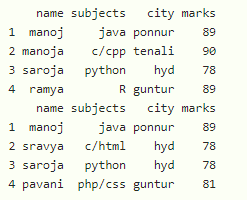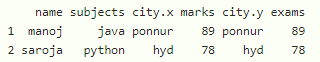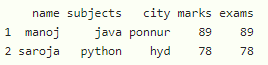Open in App
Not now

# How to Merge DataFrames Based on Multiple Columns in R?

• Last Updated : 28 Nov, 2021

In this article, we will discuss how to merge dataframes based on multiple columns in R Programming Language.

We can merge two  dataframes based on multiple columns  by using merge() function

Syntax:

merge(dataframe1, dataframe2, by.x=c(‘column1’, ‘column2’………..,’column n’), by.y=c(‘column1’, ‘column2’………..,’column n’))

where

• dataframe1 is the first dataframe
• dataframe2 is the second dataframe
• by.x represents first dataframe columns
• by.y represents second dataframe columns

Let create two dataframes and display

Example: R program to create two dataframes with 4 columns of student data

## R

 `# create dataframe1``data1 = ``data.frame``(name=``c``(``"manoj"``, ``"manoja"``, ``"saroja"``, ``"ramya"``),``                   ``subjects=``c``(``"java"``, ``"c/cpp"``, ``"python"``, ``"R"``),``                   ``city=``c``(``"ponnur"``, ``"tenali"``, ``"hyd"``, ``"guntur"``),``                   ``marks=``c``(89, 90, 78, 89))`` ` `# create dataframe2``data2 = ``data.frame``(name=``c``(``"manoj"``, ``"sravya"``, ``"saroja"``, ``"pavani"``),``                   ``subjects=``c``(``"java"``, ``"c/html"``, ``"python"``, ``"php/css"``),``                   ``city=``c``(``"ponnur"``, ``"hyd"``, ``"hyd"``, ``"guntur"``),``                   ``marks=``c``(89, 78, 78, 81))`` ` `# display``print``(data1)``print``(data2)`

Output:Example 1 : Merge dataframes on 2 columns

## R

 `# create dataframe1``data1 = ``data.frame``(name=``c``(``"manoj"``, ``"manoja"``, ``"saroja"``, ``"ramya"``),``                   ``subjects=``c``(``"java"``, ``"c/cpp"``, ``"python"``, ``"R"``),``                   ``city=``c``(``"ponnur"``, ``"tenali"``, ``"hyd"``, ``"guntur"``),``                   ``marks=``c``(89, 90, 78, 89))`` ` `# create dataframe2``data2 = ``data.frame``(student=``c``(``"manoj"``, ``"sravya"``, ``"saroja"``, ``"pavani"``),``                   ``subjects=``c``(``"java"``, ``"c/html"``, ``"python"``, ``"php/css"``),``                   ``city=``c``(``"ponnur"``, ``"hyd"``, ``"hyd"``, ``"guntur"``),``                   ``exams=``c``(89, 78, 78, 81))`` ` `# merge dataframes based on name and subjects from data1``# with student and subjects from data2``merge``(data1, data2, by.x=``c``(``'name'``, ``'subjects'``),``      ``by.y=``c``(``'student'``, ``'subjects'``))`

Output:Example 2: Merge the dataframes based on three columns.

## R

 `# create dataframe1``data1 = ``data.frame``(name=``c``(``"manoj"``, ``"manoja"``, ``"saroja"``, ``"ramya"``),``                   ``subjects=``c``(``"java"``, ``"c/cpp"``, ``"python"``, ``"R"``),``                   ``city=``c``(``"ponnur"``, ``"tenali"``, ``"hyd"``, ``"guntur"``),``                   ``marks=``c``(89, 90, 78, 89))`` ` `# create dataframe2``data2 = ``data.frame``(student=``c``(``"manoj"``, ``"sravya"``, ``"saroja"``, ``"pavani"``),``                   ``subjects=``c``(``"java"``, ``"c/html"``, ``"python"``, ``"php/css"``),``                   ``city=``c``(``"ponnur"``, ``"hyd"``, ``"hyd"``, ``"guntur"``),``                   ``exams=``c``(89, 78, 78, 81))`` ` `# merge dataframes based on name,city and subjects from data1``# with student,city and subjects from data2``merge``(data1, data2, by.x=``c``(``'name'``, ``'subjects'``, ``'city'``),``      ``by.y=``c``(``'student'``, ``'subjects'``, ``'city'``))`

Output:My Personal Notes arrow_drop_up